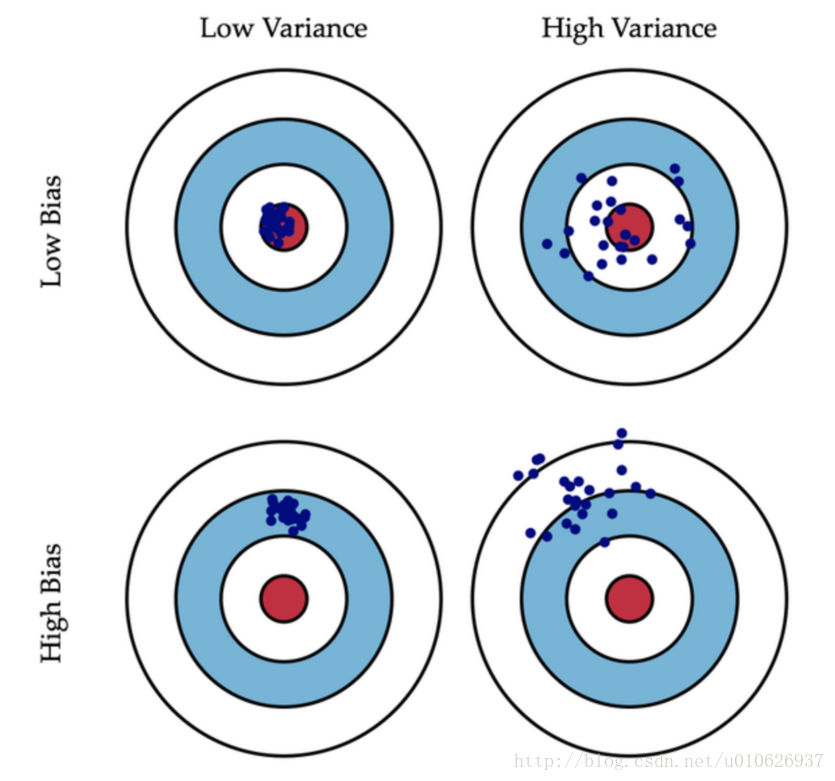2018-12-10 22:39:29 feilong_csdn 阅读数 417
• ###### 机器学习之概率与统计推断

本课程讲解机器学习算法所需概率和统计推断知识。概率部分包括概率公理及推论、条件概率、贝叶斯公式、随机变量及其概率函数（CDF／pdf）、常用概率分布及其均值、方差；统计推断部分包括大数定律和中心极限定理、极大似然估计、贝叶斯估计，估计的评价、偏差-方差平衡。课程还会讲解假设检验的基本概念。

20155 人正在学习 去看看 AI100讲师

## 一、理解偏差、方差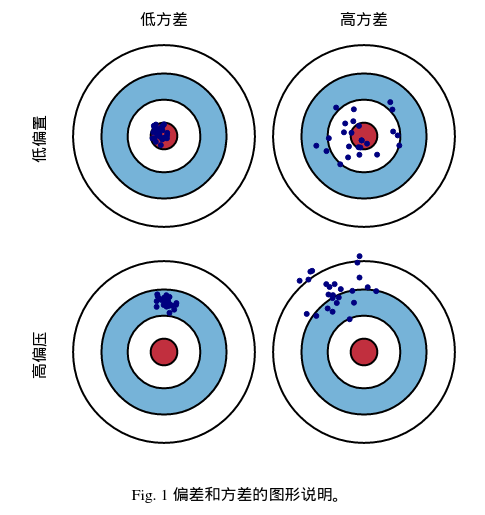## 二、偏差、方差与模型范化能力

$\text{Err}\left( x \right) = \ \text{bias}^{2}\left( x \right) + var\left( x \right) + \ \varepsilon^{2}$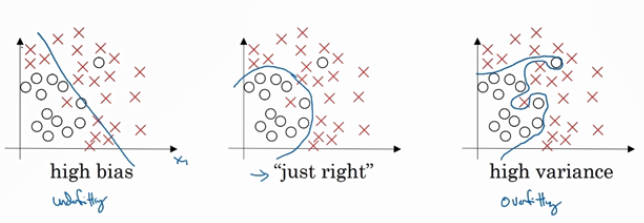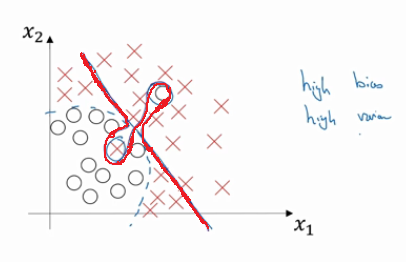$\text{Err}\left( x \right) = \ \text{bias}^{2}\left( x \right) + var\left( x \right) + \ \varepsilon^{2}$

x：测试样本集

D：训练数据集

yD：测试数据集x的标记

y：测试数据集x的真实标记

f：由训练集D学习到的模型

f(x,D)：模型f对x的预测输出

f(x)：模型f对x预测输出的均值，即期望预测输出

$\overset{\overline{}}{f}\left( x \right) = E(f(x,D))$

$\text{bias}^{2}\left( x \right) = \ \left( \overset{\overline{}}{f}\left( x \right) - y \right)^{2}$

$\text{var}\left( x \right) = \ {E\left\lbrack f\left( x,D \right) - \ \overset{\overline{}}{f}\left( x \right) \right\rbrack}^{2}$

$\varepsilon^{2} = E\left\lbrack \left( y_{D} - y \right)^{2} \right\rbrack$

$E\left\lbrack y_{D} - y \right\rbrack = 0$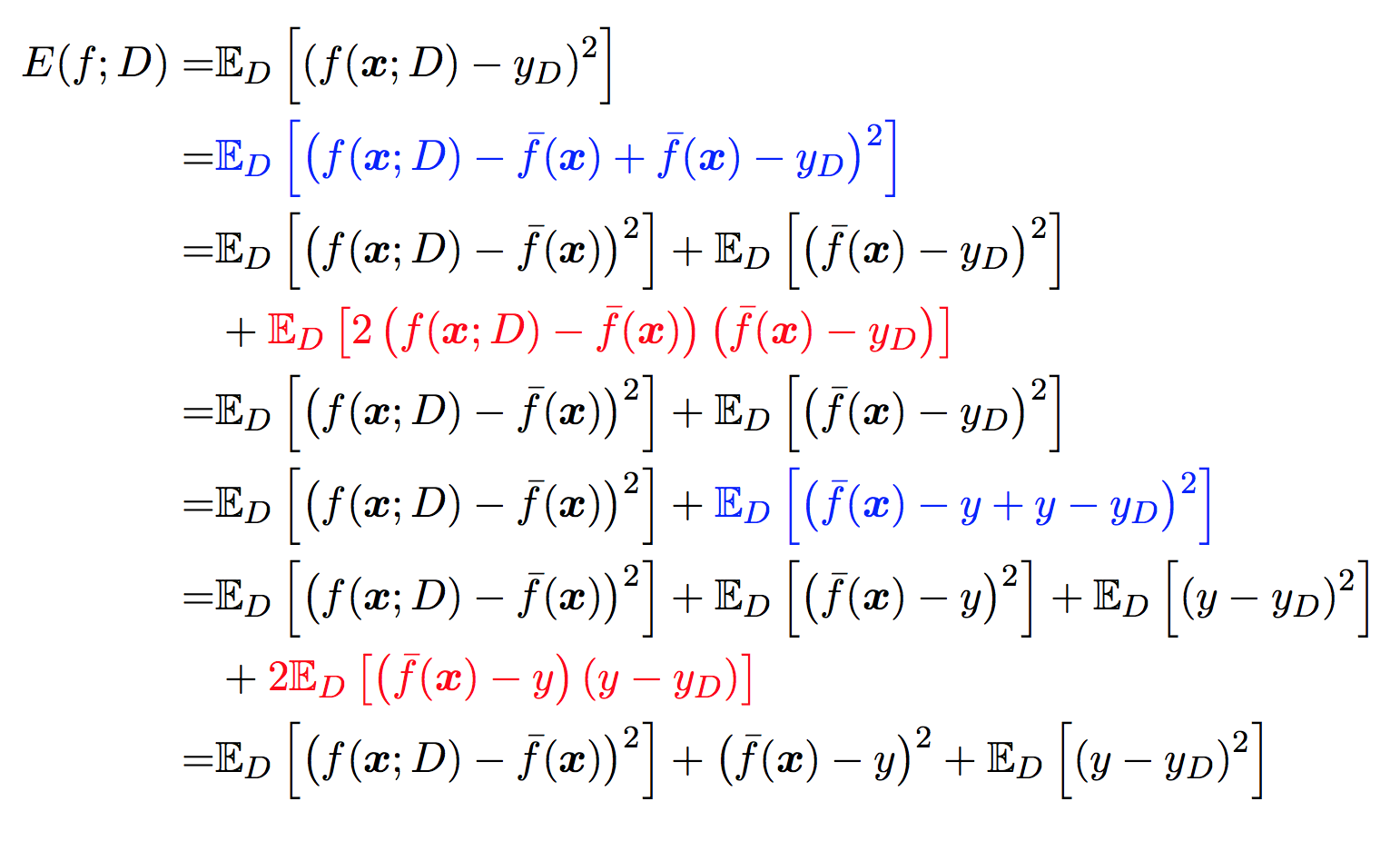$E\left\lbrack f\left( x,D \right) - \ \overset{\overline{}}{f}\left( x \right) \right\rbrack = 0$

$E\left\lbrack y_{D} - y \right\rbrack = 0$

$E_{D}\left( 2\left( f\left( x,D \right) - \overset{\overline{}}{f}\left( x \right) \right)\left( \overset{\overline{}}{f}\left( x \right) - y_{D} \right) \right) = 0$

$E_{D}\left( (\overset{\overline{}}{f}\left( x \right) - y)\left( y - y_{D} \right) \right) = 0$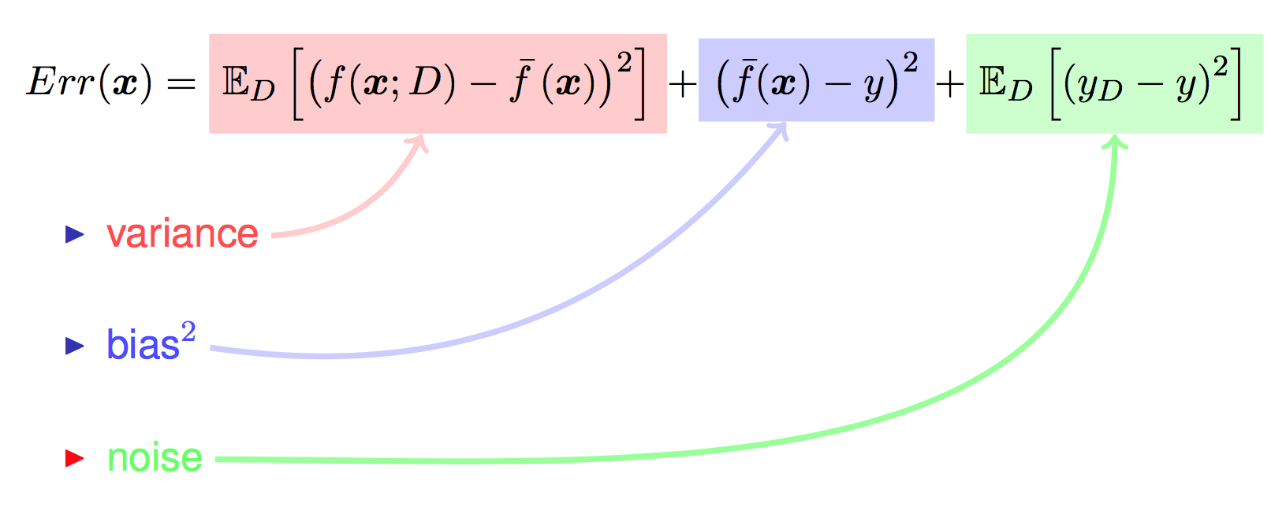$\text{Err}\left( x \right) = \ \text{bias}^{2}\left( x \right) + var\left( x \right) + \ \varepsilon^{2}$

## 三、模型偏差高 or 方差高

(1)训练集错误率：1%、测试集错误率：11% 低偏差高方差

(2)训练集错误率：15%、测试集错误率：16% 高偏差低方差

(3)训练集错误率：15%、测试集错误率：30% 高偏差高方差

(4)训练集错误率：0.5%、测试集错误率：1% 低偏差低方差

## 四、高偏差高方差时优化模型

##### 1、高偏差(模型欠拟合)时模型优化方法

(1)添加特征数

(2)增加模型复杂度

(3)延长训练时间

(4)减小正则化系数

(5)集成学习方法Boosting
Boosting算法是将多个弱分类串联在一起，如Boosting算法训练过程中，我们计算弱分类器的错误和残差，作为下一个分类器的输入，这个过程本身就在不断减小损失函数，减小模型的偏差

(6)选用更合适的模型

##### 2、高方差(模型过拟合)时模型优化方法

(1)增加数据集

(2)降低模型的复杂度

(3)正则化防止过拟合

$C\left( x \right) = \ \frac{1}{2}\sum_{i = 1}^{n}{(f\left( x \right) - y_{i})}^{2}$

$C\left( x \right) = \ \frac{1}{2}\sum_{i = 1}^{n}{{(f\left( x \right) - y_{i})}^{2} + \ \lambda L}$

<1> L1正则化(L1范数)：权重向量w的绝对值之和

$L_{1} = \ \sum_{i = 1}^{k}{||w_{i}||}_{1}$

<2> L2正则化(L2范数)：权重向量w的平方和，欧几里得范数

$L_{2} = \ \sum_{i = 1}^{k}{||w_{i}||}_{2}^{2}$

(4)集成学习方法Bagging

(5)选用更合适的模型

## 五、谈偏差和Boosting、方差和Bagging

##### 1、Bagging和方差

Bagging算法对数据重采样，然后在每个样本集训练出来的模型上取平均值

$var = \ n^{2}*\gamma^{2}*\delta^{2}*\rho + m*\gamma^{2}*\delta^{2}*(1 - \rho)$

$\ \ \ \ \ \ \ = n^{2}*\frac{1}{n^{2}}*\delta^{2}*\rho + m*\frac{1}{n^{2}}*\delta^{2}*(1 - \rho)$

$= \rho*\delta^{2} + \left( 1 - \rho \right)*\frac{\delta^{2}}{n}\text{\ \ \ \ \ \ \ \ \ \ \ \ \ \ \ \ \ \ \ \ \ \ \ \ \ \ \ \ }$

Bagging算法对n个独立不相关的模型的预测结果取平均，方差是原来单个模型1/n，上述描述不严谨因为在实际问题中，模型不可能完全独立，但为了追求模型的独立性，Bagging的方法做了不同的改进，比如随机森林算法中，每次选取节点分裂属性时，会随机抽取一个属性子集，而不是从所有的属性中选最有属性，这就为了避免弱分类器之间过强的关联性，通过训练集的重采样也能够带来弱分类器之间的一定独立性，这样多个模型学习数据，不会因为一个模型学习到数据某个特殊特性而造成方差过高

##### 2、Boosting和偏差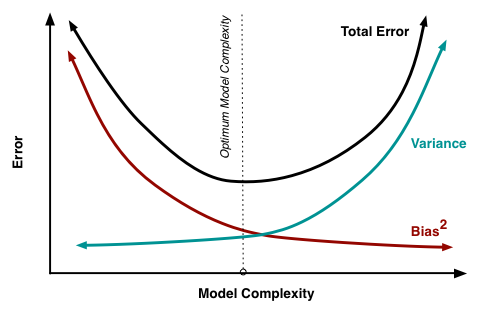##### 1、偏差方差权衡之经验风险最小化模型

$\text{Error}_{\text{train}} = \text{Error}_{\text{test}}$

$\text{Error}_{\text{train}}\ \approx \ \ 0$

(1)联合界引理

$P\left( A_{1}\bigcup..\bigcup A_{k} \right) \leq P\left( A_{1} \right) + P\left( A_{2} \right) + \ldots + P(A_{k})$

(2)Hoeffding不等式
Z1,Z2,…,Zm是m个独立同分布的随机变量，他们服从均值为φ的伯努利分布(0-1分布)，即表示P(Zi=1)=φ，所有对m个独立同分布的随机变量，其均值为φ，给定λ > 0

$P\left( \left| \phi - \hat{\phi} \right| > \gamma \right) \leq 2exp( - 2\gamma^{2}m)$

https://blog.csdn.net/zb123455445/article/details/78538361

##### 2、偏差方差权衡之交叉验证

(1) Hold-out cross validation 保留交叉验证

(2)留1交叉验证

(3)K重交叉验证

2016-08-07 15:47:00 duxinyuhi 阅读数 655
• ###### 机器学习之概率与统计推断

本课程讲解机器学习算法所需概率和统计推断知识。概率部分包括概率公理及推论、条件概率、贝叶斯公式、随机变量及其概率函数（CDF／pdf）、常用概率分布及其均值、方差；统计推断部分包括大数定律和中心极限定理、极大似然估计、贝叶斯估计，估计的评价、偏差-方差平衡。课程还会讲解假设检验的基本概念。

20155 人正在学习 去看看 AI100讲师S为样本方差，样本方差除以n-1而不是n，是因为样本可能没有全部反映总体，除以n-1 比除以n2019-05-28 16:31:34 meijie2018_1 阅读数 208
• ###### 机器学习之概率与统计推断

本课程讲解机器学习算法所需概率和统计推断知识。概率部分包括概率公理及推论、条件概率、贝叶斯公式、随机变量及其概率函数（CDF／pdf）、常用概率分布及其均值、方差；统计推断部分包括大数定律和中心极限定理、极大似然估计、贝叶斯估计，估计的评价、偏差-方差平衡。课程还会讲解假设检验的基本概念。

20155 人正在学习 去看看 AI100讲师

1、偏差与方差的概念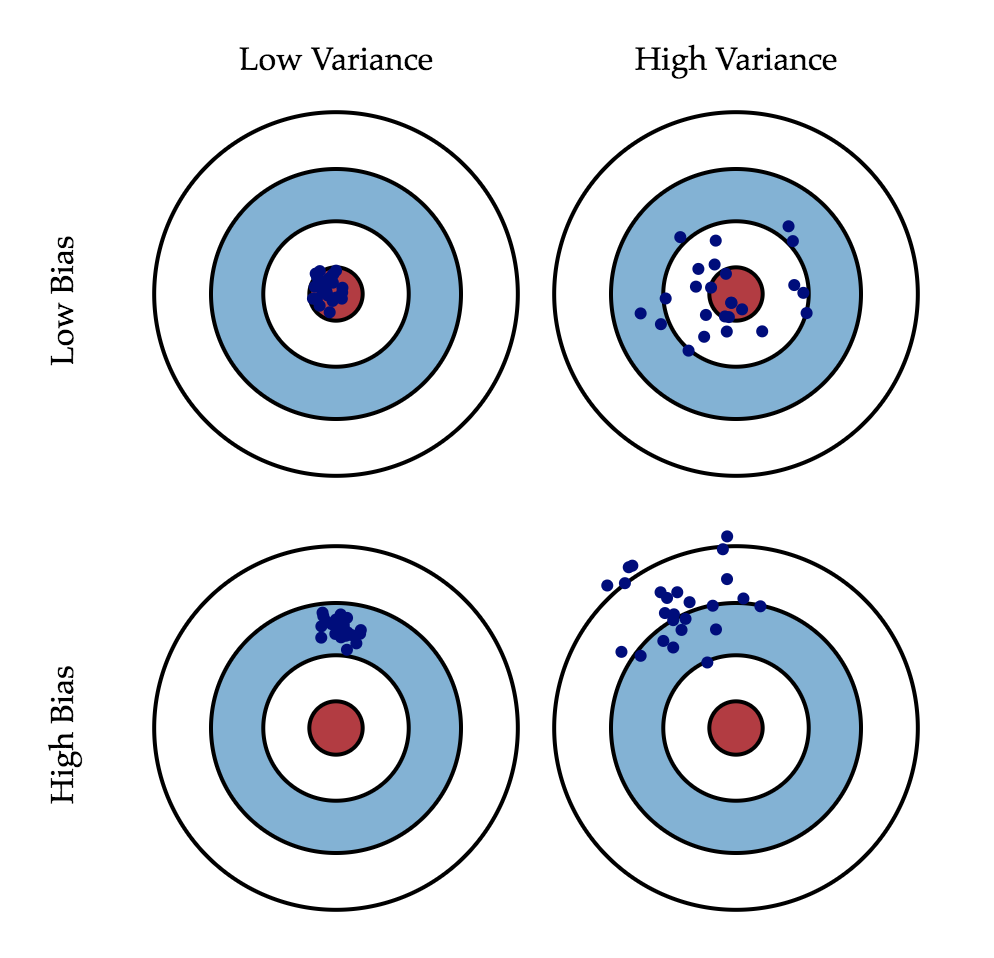2、过拟合与欠拟合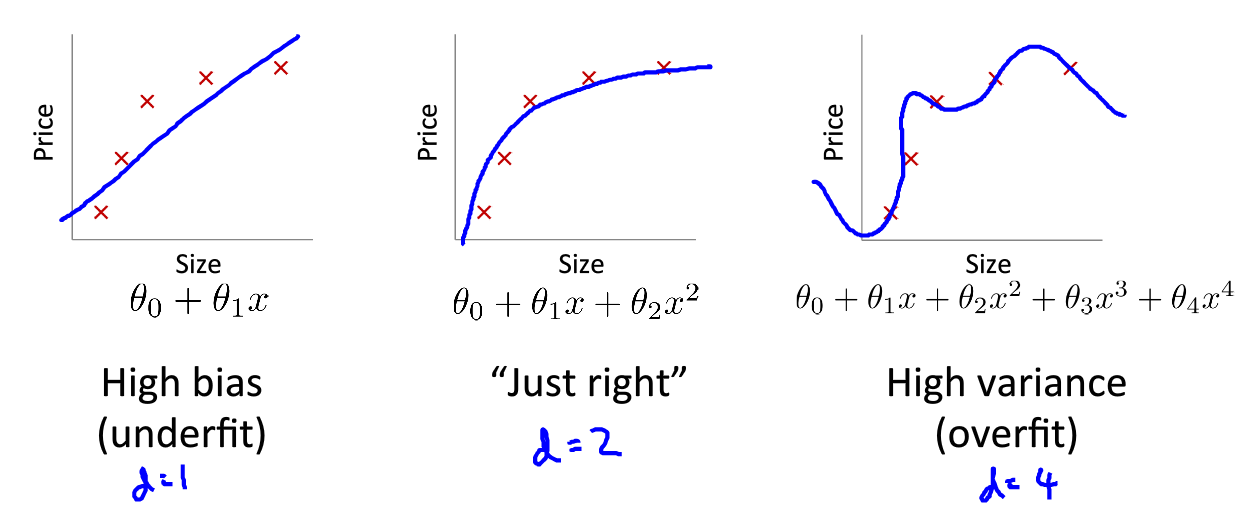3、两者之间的关系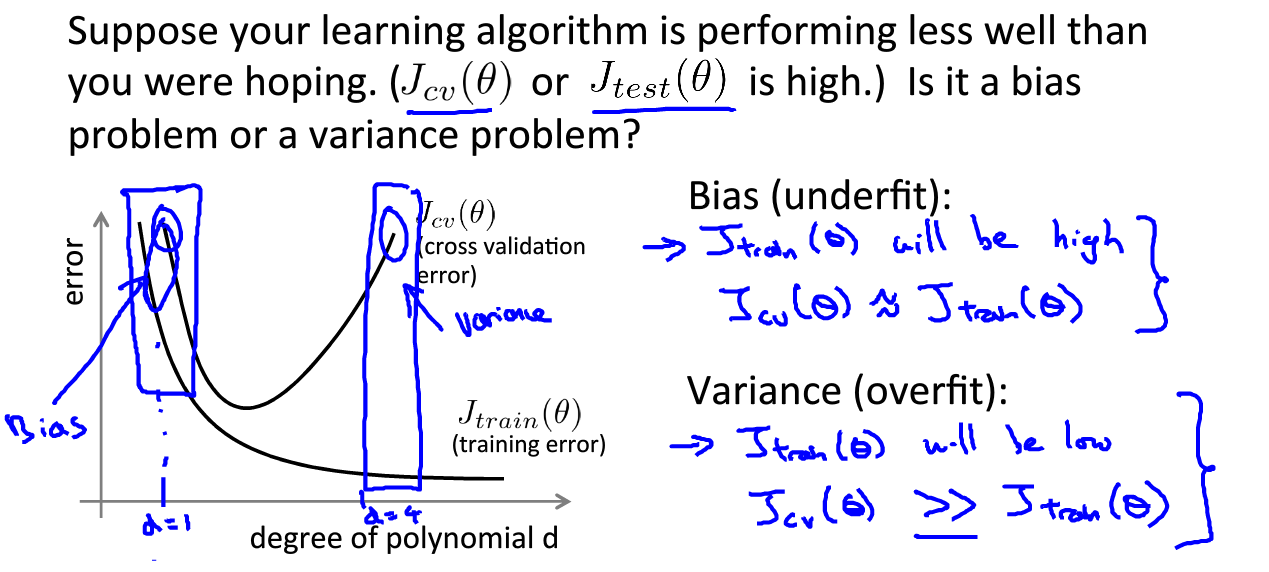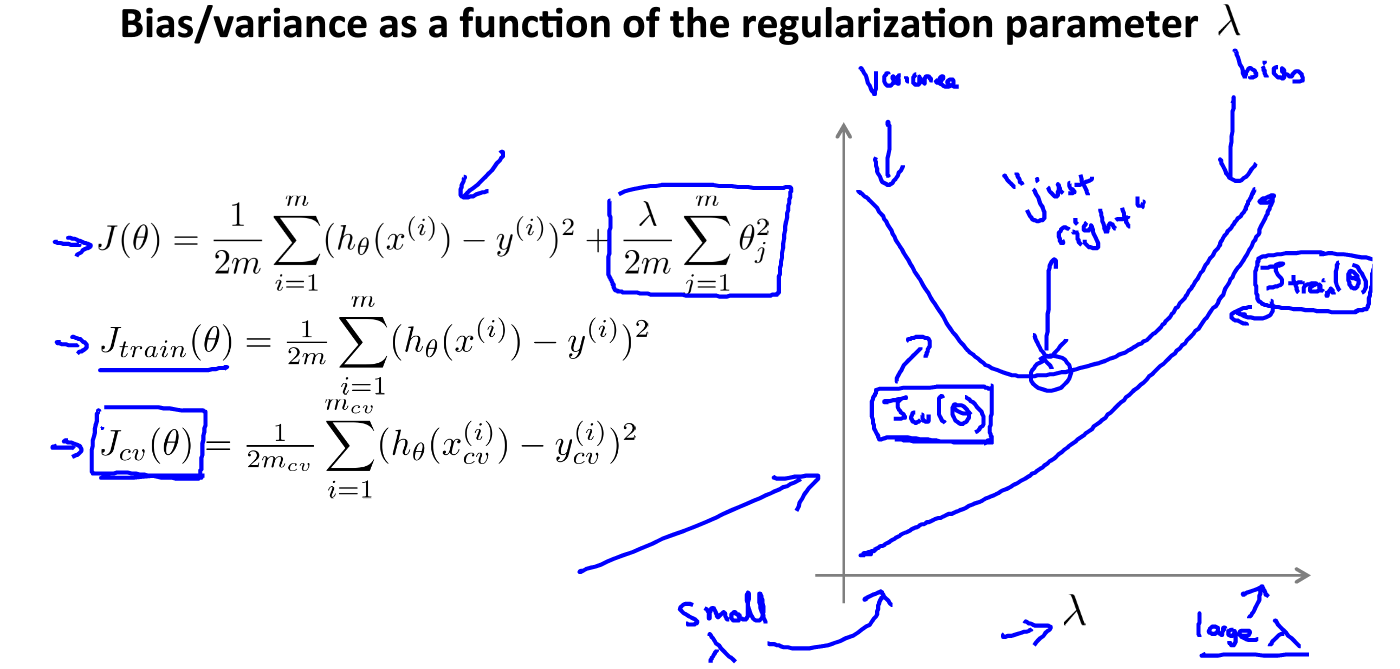2017-08-07 20:32:10 liweibin1994 阅读数 4677
• ###### 机器学习之概率与统计推断

本课程讲解机器学习算法所需概率和统计推断知识。概率部分包括概率公理及推论、条件概率、贝叶斯公式、随机变量及其概率函数（CDF／pdf）、常用概率分布及其均值、方差；统计推断部分包括大数定律和中心极限定理、极大似然估计、贝叶斯估计，估计的评价、偏差-方差平衡。课程还会讲解假设检验的基本概念。

20155 人正在学习 去看看 AI100讲师2019-03-30 22:24:30 u012966380 阅读数 79
• ###### 机器学习之概率与统计推断

本课程讲解机器学习算法所需概率和统计推断知识。概率部分包括概率公理及推论、条件概率、贝叶斯公式、随机变量及其概率函数（CDF／pdf）、常用概率分布及其均值、方差；统计推断部分包括大数定律和中心极限定理、极大似然估计、贝叶斯估计，估计的评价、偏差-方差平衡。课程还会讲解假设检验的基本概念。

20155 人正在学习 去看看 AI100讲师

1.寻找更好的特征（可以先使用gdbt筛选一波）
2.用更多的特征 （增加数据维度）

1.增大训练数据集合 （增加训练数据量）
2.减少训练数据特征（减小数据维度）
3.尝试增加正则化程度